# Lagrange interpolation formula

Jump to: navigation, search

A formula for obtaining a polynomial of degree(the Lagrange interpolation polynomial) that interpolates a given functionat nodes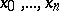:(1)

When theare equidistant, that is,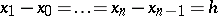, using the notation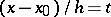one can reduce (1) to the form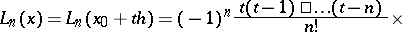(2)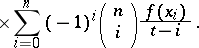In the expression (2), called the Lagrange interpolation formula for equidistant nodes, the coefficientsof the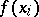are called the Lagrange coefficients.

Ifhas a derivative of orderon the interval, if all interpolation nodes lie in this interval and if for any point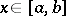one defines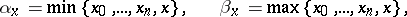then a point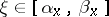exists such that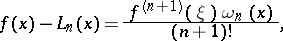where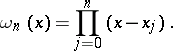If the absolute value of the derivative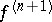is bounded onby a constantand if the interpolation nodes are chosen such that the roots of the Chebyshev polynomial of degreeare mapped into these points under a linear mapping fromonto, then for anyone has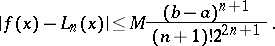If the interpolation nodes are complex numbers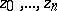and lie in some domainbounded by a piecewise-smooth contour, and ifis a single-valued analytic function defined on the closure of, then the Lagrange interpolation formula has the formwhereThe Lagrange interpolation formula for interpolation by means of trigonometric polynomials is: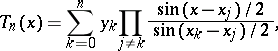which is a trigonometric polynomial of orderhaving prescribed values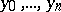at the given nodes.

The formula was proposed by J.L. Lagrange in 1795.